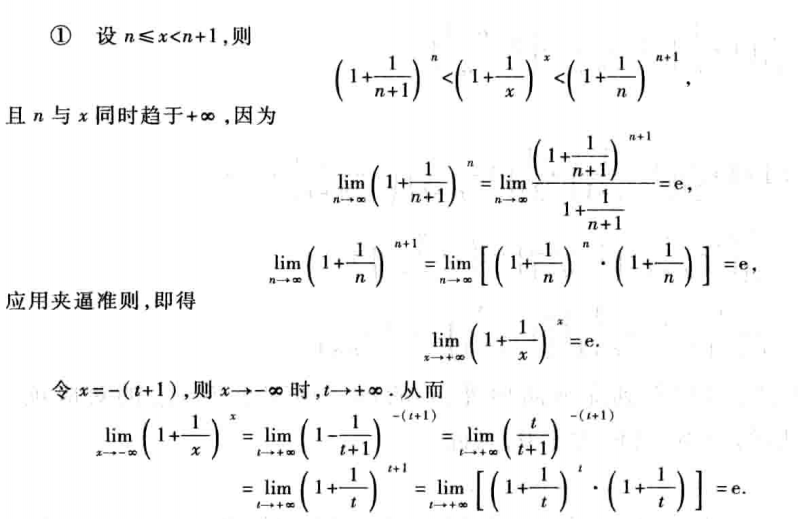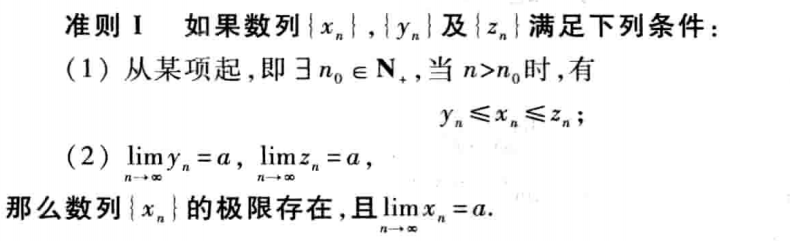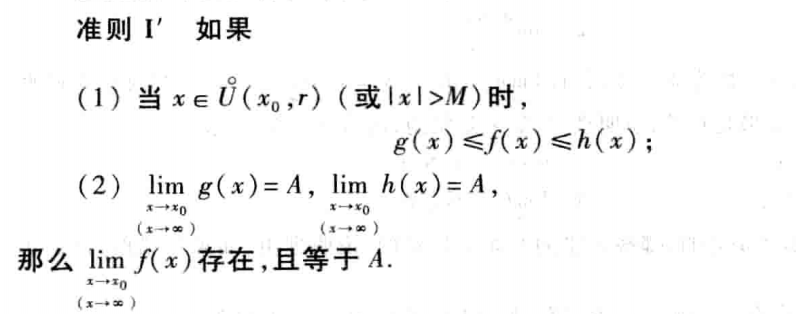特殊极限lim(x→∞)(1+1/x)^x的证明

2020/03/16 19:10而夹逼定理为下面的两个准则：lim(x→∞)g(x)=lim(x→∞)[1+1/([x]+1)]^[x]，因n=[x]，则当x→∞，n→∞，故运用复合函数的极限运算法则知，

lim(x→∞)g(x)=lim(x→∞)[1+1/([x]+1)]^[x]=lim(n→∞)[1+1/(n+1)]^n=e，

lim(x→∞)h(x)=lim(x→∞)[1+1/[x]]^([x]+1)=lim(n→∞)(1+1/n)^(n+1)=e，

0
0 收藏

作者的其它热门文章0 评论
0 收藏
0